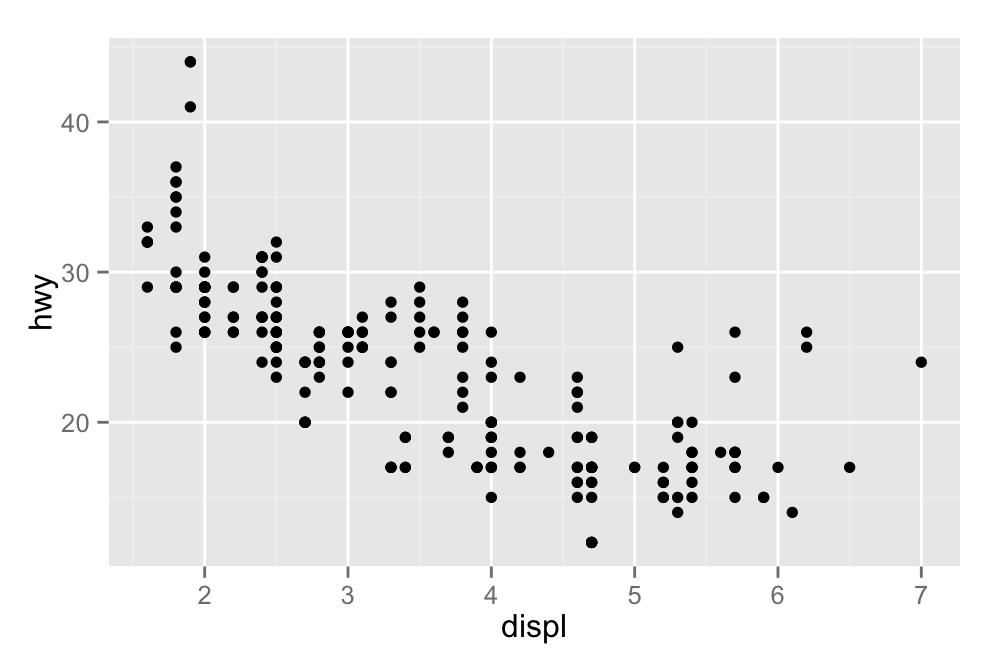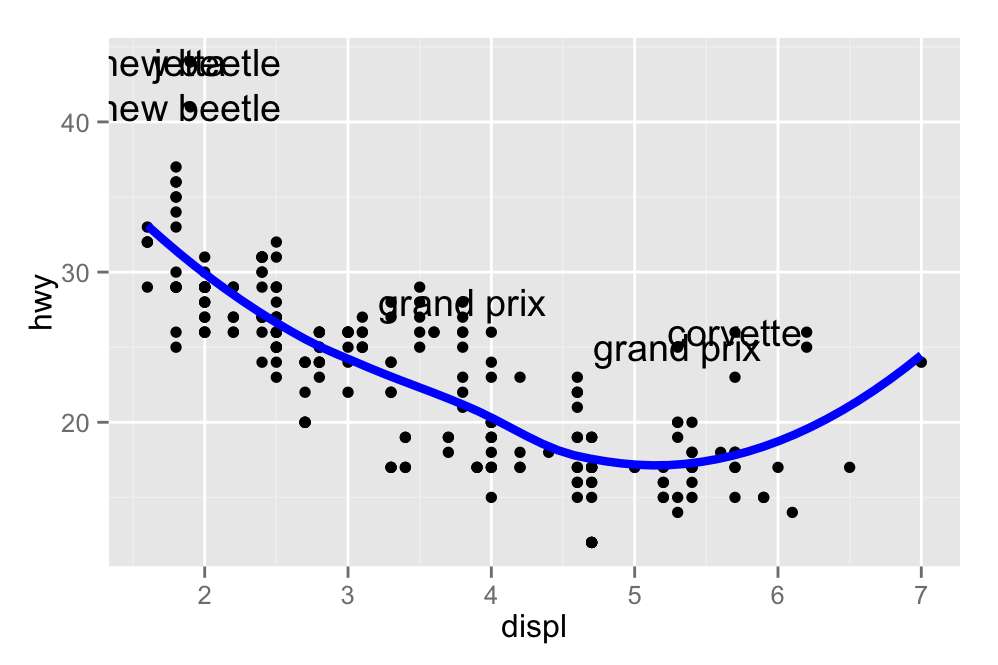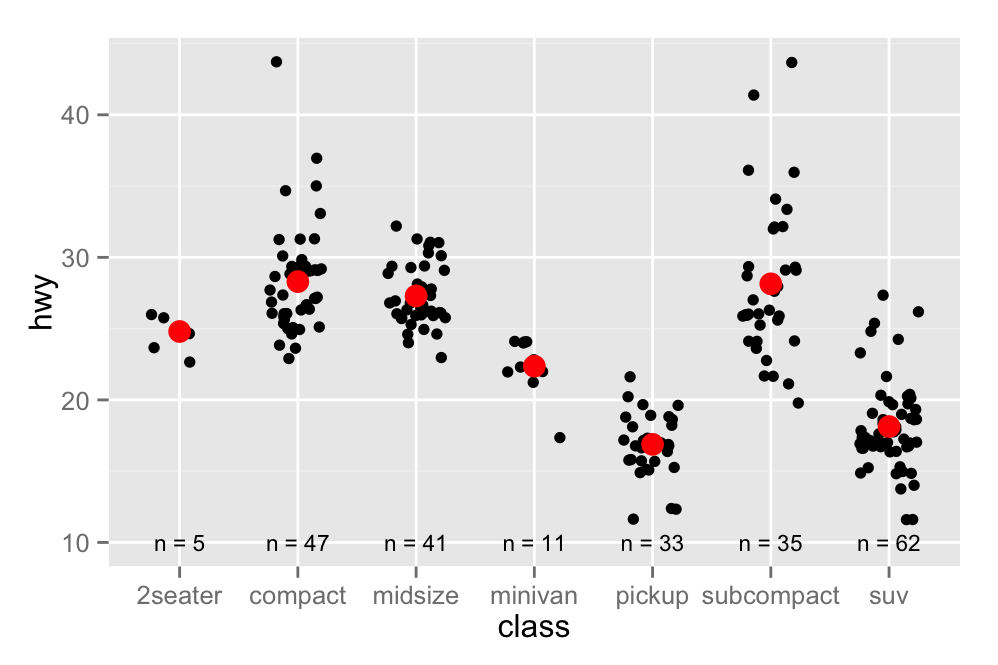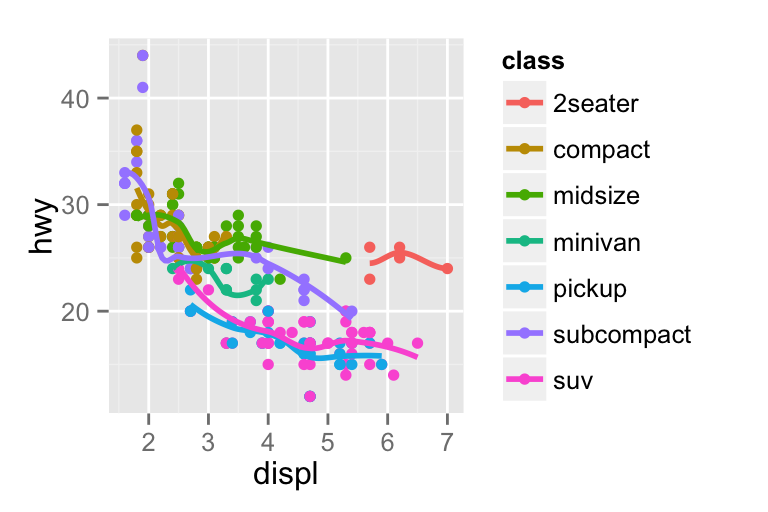# Build a plot layer by layer

## Introduction

One of the key ideas behind ggplot2 is that it allows you to easily iterate, building up a complex plot a layer at a time. Each layer can come from a different dataset and have a different aesthetic mappings, making it possible to create sophisticated plots that display data from multiple sources.

You’ve already created layers with functions like `geom_point()` and `geom_histogram()`. In this chapter, you’ll dive into the details of a layer, and how you can control all five components: data, the aesthetic mappings, the geom, stat, and position adjustments. The goal here is to give you the tools to build sophisticated plots tailored to the problem at hand. This more theoretical chapter is accompanied by the next chapter, the “toolbox”, which is more hands on, applying the basic components of a layer to specific visualisation challenges.

## Building a plot

So far, whenever we’ve created a plot with `ggplot()`, we’ve immediately added on a layer with a geom function. But it’s important to realise that there really are two distinct steps. First we create a plot with default dataset and aesthetic mappings:

``````p <- ggplot(mpg, aes(displ, hwy))
p
#> Error: No layers in plot``````

The plot can’t be displayed until we add a layer: there is nothing to see!

``p + geom_point()```geom_point()` is a shortcut. Behind the scenes it calls the `layer()` function to create a new layer:

``````p + layer(
mapping = NULL,
data = NULL,
geom = "point", geom_params = list(),
stat = "identity", stat_params = list(),
position = "identity"
)``````

This call fully specifies the five components to the layer:

• mapping: A set of aesthetic mappings, specified using the `aes()` function and combined with the plot defaults as described in aesthetic mappings. If `NULL`, uses the default mapping set in `ggplot()`.

• data: A dataset which overrides the default plot dataset. It is usually omitted (set to `NULL`), in which case the layer will use the default data specified in `ggplot()`. The requirements for data are explained in more detail in data.

• geom: The name of the geometric object to use to draw each observation. Geoms are discussed in more detail in geom, and the toolbox explores their use in more depth.

Geoms can have additional arguments. All geoms take aesthetics as parameters. If you supply an aesthetic (e.g. colour) as a parameter, it will not be scaled, allowing you to control the appearance of the plot, as described in setting vs. mapping. You can pass params in `...` (in which case stat and geom parameters are automatically teased apart), or in a list passed to `geom_params`.

• stat: The name of the statistical tranformation to use. A statistical transformation performs some useful statistical summary is key to histograms and smoothes. To keep the data as is, use the “identity” stat. Learn more in statistical transformations.

You only need to set one of stat and geom: every geom has a default stat, and every stat a default geom.

Most stats take additional parameters to specify the details of statistical transformation. You can supply params either in `...` (in which case stat and geom parameters are automatically teased apart), or in a list called `stat_params`.

• position: The method used to adjusting overlapping objects, like jittering, stacking or dodging. More details in position.

It’s useful to understand the `layer()` function so you have a better mental model of the layer object. But you’ll rarely use the full `layer()` call because it’s so verbose. Instead, you’ll use the shortcut `geom_` functions: `geom_point(mapping, data, ...)` is exactly equivalent to `layer(mapping, data, geom = "point", ...)`.

## Data

Every layer must have some data associated with it, and that data must be in a data frame. This is a strong restriction, but there are good reasons for it:

• Your data is very important, and it’s best to be explicit about it.

• A single data frame is also easier to save than a multitude of vectors, which means it’s easier to reproduce your results or send your data to someone else.

• It enforces a clean separation of concerns: ggplot2 turns data frames into visualisations. Other packages can make data frames in the right format (learn more about that in model visualisation).

The data on each layer doesn’t need to be the same, and it’s often useful to combine multiple datasets in a single plot. To illustrate that idea I’m going to generate two new datasets related to the mpg dataset. First I’ll fit a loess model and generate predictions from it. (This is what `geom_smooth()` does behind the scenes)

``````mod <- loess(hwy ~ displ, data = mpg)
grid <- data.frame(displ = seq(min(mpg\$displ), max(mpg\$displ), length = 50))
grid\$hwy <- predict(mod, newdata = grid)

#>   displ  hwy
#> 1  1.60 33.1
#> 2  1.71 32.2
#> 3  1.82 31.3
#> 4  1.93 30.4
#> 5  2.04 29.6
#> 6  2.15 28.8``````

Next, I’ll isolate observations that are particularly far away from their predicted values:

``````std_resid <- resid(mod) / mod\$s
outlier <- subset(mpg, abs(std_resid) > 2)``````

I’ve generated these datasets because it’s common to enhance the display of raw data with a statistical summary and some annotations. With these new datasets, I can improve our initial scatterplot by overlaying a smoothed line, and labelling the outlying points:

``````ggplot(mpg, aes(displ, hwy)) +
geom_point() +
geom_line(data = grid, colour = "blue", size = 1.5) +
geom_text(data = outlier, aes(label = model))``````(The text labels aren’t particularly easy to read, but you’ll learn how to improve those in [polishing]{#cha:polishing}.)

In this example, every layer uses a different dataset. We could define the same plot in another way, omitting the default dataset:

``````ggplot(mapping = aes(displ, hwy)) +
geom_point(data = mpg) +
geom_line(data = grid) +
geom_text(data = outlier, aes(label = model))``````

For this case, I don’t particularly like this style because it makes it less clear what the primary dataset is (and because of the way that the arguments to `ggplot()` are ordered, it actually requires more keypresses!). However, you may prefer it in cases where there isn’t a clear primary dataset, or where the aesthetics also vary from layer to layer.

NB: if you omit the data set in the call to `ggplot()` you must explicitly supply a dataset for every layer. Also note that facetting will not work without a default dataset: faceting affects all layers so it needs to have a base dataset that defines the set of facets. See missing faceting variables for more details.

### Exercises

1. The first two arguments to ggplot are `data` and `mapping`. The first two arguments to all layer functions are `mapping` and `data`. Why does the order of the arguments differ? (Hint: think about what you set most commonly.)

2. The following code uses dplyr to generate some summary statistics about each class of car (you’ll learn how it works in data transformation).

``````library(dplyr)
class <- mpg %>%
group_by(class) %>%
summarise(n = n(), hwy = mean(hwy))``````

Use the data to recreate this plot:## Aesthetic mappings

The aesthetic mappings, defined with `aes()`, describe how variables are mapped to visual properties or aesthetics. `aes()` takes a sequence of aesthetic-variable pairs like this:

``aes(x = displ, y = hwy, colour = class)``

(If you’re American, you can use color, and behind the scenes ggplot2 will correct your spelling ;)

Here we map x-position to `displ`, y-position to `hwy`, and colour to `class`. The names for the first two arguments can be ommitted, in which case they correspond to the x and y variables. That makes this specification equivalent to the one above:

``aes(displ, hwy, colour = class)``

While you can do data manipulation in `aes()`, e.g. `aes(log(carat), log(price))`, best to only do simple calculations. It’s better to move complex transformations out of `aes()` call and into an explicit `mutate()` call, as you’ll learn about in mutate. This makes it easier to check your work and it’s often faster (because you need only do the transformation once, not every time the plot is drawn).

Avoid referring to variables that are not in the data (e.g., with `diamonds\$carat`). This breaks containment, so that the plot no longer contains everything it needs. This model is a slight simplification: every ggplot has an environment associated with it, so you can refer to objects in that environment and it will work. However, it’s best not to rely on this as it prevents the plot from being self-contained. ggplot2 was written before I fully understood non-standard evaluation in R, so it’s not as reliable as it could be.

### Specifying the aesthetics in the plot vs. in the layers

Aesthetic mappings can be supplied in the initial `ggplot()` call, in individual layers, or in some combination of both. All of these calls create the same plot specification:

``````ggplot(mpg, aes(displ, hwy, colour = class)) +
geom_point()
ggplot(mpg, aes(displ, hwy)) +
geom_point(aes(colour = class))
ggplot(mpg, aes(displ)) +
geom_point(aes(y = hwy, colour = class))
ggplot(mpg) +
geom_point(aes(displ, hwy, colour = class))``````

You can add, override, or remove mappings:

Operation Layer aesthetics Result
Add `aes(colour = cyl)` `aes(mpg, wt, colour = cyl)`
Override `aes(y = disp)` `aes(mpg, disp)`
Remove `aes(y = NULL)` `aes(mpg)`

If you only have one layer in the plot, the way you specify aesthetics doesn’t make any matter. However, the distinction is important when you start adding additional layers. These two plots are both valid and interesting, but focus on quite different aspects of the data:

``````ggplot(mpg, aes(displ, hwy, colour = class)) +
geom_point() +
geom_smooth(se = FALSE)

ggplot(mpg, aes(displ, hwy)) +
geom_point(aes(colour = class)) +
geom_smooth(se = FALSE)``````